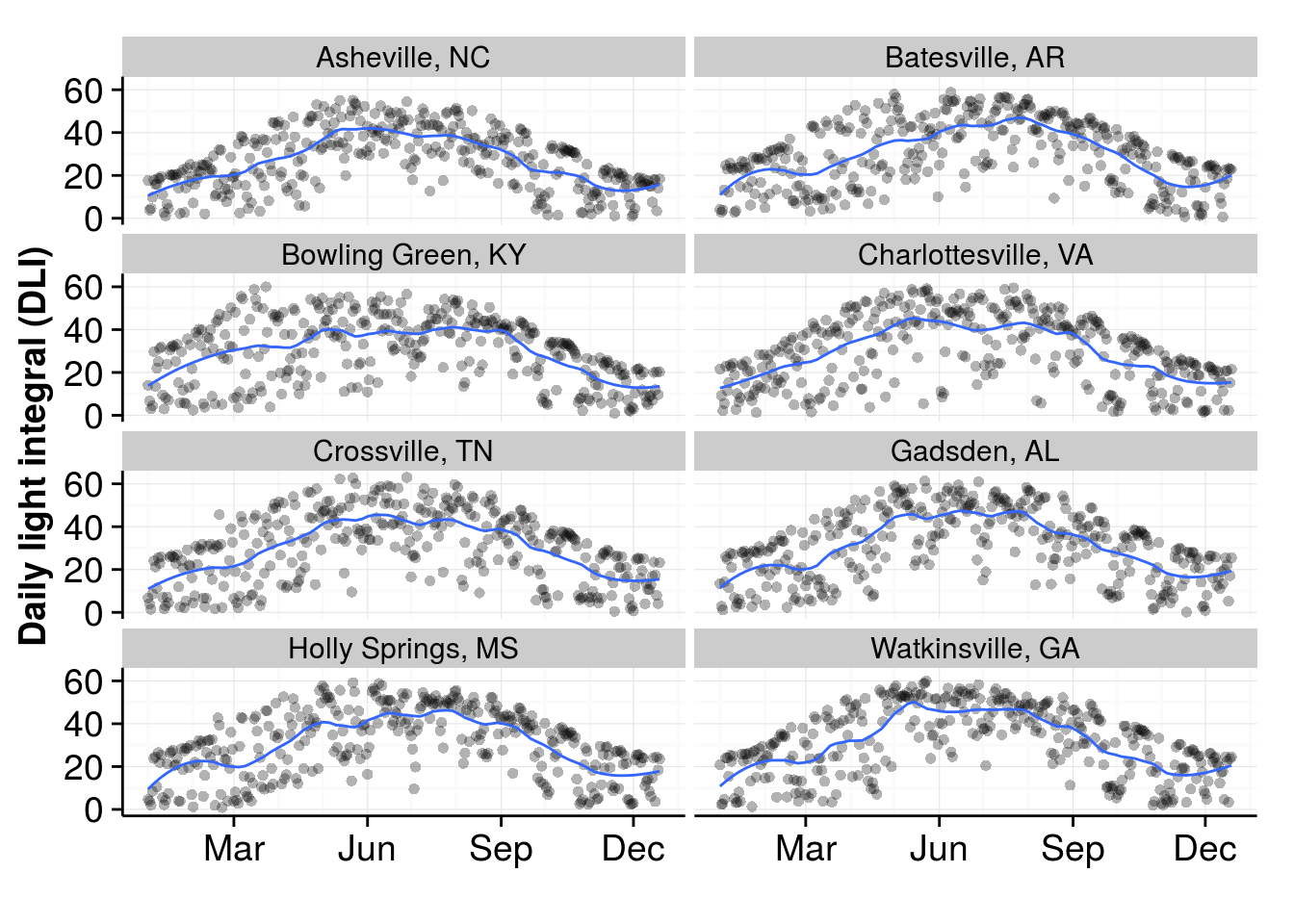This describes how I estimated the daily light integral (DLI) for 2015 in Chattanooga, Knoxville, Memphis, and Nashville. The code for this document, and for the calculations, is at https://github.com/micahwoods/dli_tn.

I had written about an average DLI of 40 being a no problem level for bermudagrass putting greens. Brandon Horvath asked if I could generate some DLI values for Tennessee locations.

That sound like it would be easy, but it takes a bit of calculation. It’s not like there are sensors for photosynthetically active radiation (PAR) all around the state, streaming the measurements or making them available for download. If there are such data sources, please let me know!

Here’s what I did.

### Step 1, get measured data from nearby stations

I knew that DLI data for the 4 cities of interest were not available, but I could get global solar radiation (Rs) measurements for Crossville, TN, and for a number of locations in the surrounding states. nrcc_nearby_stations.R gets the 2015 daily summaries from the U.S. Climate Reference Network (Diamond et al. 2013). Then, I converted the measured Rs from units of MJ m-2 d-1 to DLI in units of moles m-2 d-1. I did this by multiplying Rs by 2.04, the factor given in Meek et al. (1984).

Then I plotted the DLI for these locations in 2015, with a blue line showing a moving average of DLI.### Step 2, compare calculated DLI to measured DLI

For the four cities in Tennessee, I would need to calculate the DLI. Before doing that, I wanted to check if the calculated DLI was similar to the the measured DLI.

I used the Hargreaves radiation formula to estimate Rs, from the estimating missing radiation data section of the FAO Crop Evapotranspiration book (Allen et al. 1998).

$R_{s} = k_{R_{s}}\sqrt{T_{max} - T_{min}}R_{a}$

where

• $$R_{a}$$ is the extraterrestrial solar radiation
• $$T_{max}$$ is the maximum air temperature
• $$T_{min}$$ is the minimum air temperature
• $$k_{R_{s}}$$ is an adjustment coefficient; I used 0.16 which is the standard for interior (not coastal) locations

Now I had a measured Rs and an estimated Rs for these locations, and I calculated DLI using the same 2.04 factor, and then had a look at how the estimated Rs could predict the measured Rs.